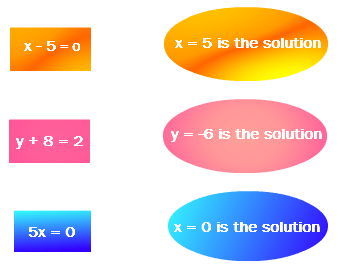# Linear Equations in One Variable worksheet for class 8## myCBSEguide App

CBSE, NCERT, JEE Main, NEET-UG, NDA, Exam Papers, Question Bank, NCERT Solutions, Exemplars, Revision Notes, Free Videos, MCQ Tests & more.

Linear Equations in One Variable worksheet for class 8

## Linear Equations in One Variable worksheet for class 8 Important Topics

• Introduction
• Solving Equations which have Linear Expressions on one Side and Numbers on the other Side
• Some Applications
• Solving Equations having the Variable on both Sides
• Some More Applications
• Reducing Equations to Simpler Form
• Equations Reducible to the Linear Form## Some important Facts about Linear Equations in One Variable worksheet for class 8

1.  An algebraic equation is an equality involving variables. It says that the value of the expression on one side of the equality sign is equal to the value of the expression on the other side.
2. The equations we study in Classes VI, VII and VIII are linear equations in one variable. In such equations, the expressions which form the equation contain only one variable. Further, the equations are linear, i.e., the highest power of the variable appearing in the equation is 1.
3. A linear equation may have for its solution any rational number.
4. An equation may have linear expressions on both sides. Equations that we studied in Classes VI and VII had just a number on one side of the equation.
5. Just as numbers, variables can, also, be transposed from one side of the equation to the other.
6. Occasionally, the expressions forming equations have to be simplified before we can solve them by usual methods. Some equations may not even be linear to begin with, but they can be brought to a linear form by multiplying both sides of the equation by a suitable expression.
7. The utility of linear equations is in their diverse applications; different problems on numbers, ages, perimeters, combination of currency notes, and so on can be solved using linear equations

## Solving Equations having the Variable on both Sides

An equation is the equality of the values of two expressions. In the equation 2x – 3 = 7, the two expressions are 2x – 3 and 7. In most examples that we have come across so far, the RHS is just a number. But this need not always be so; both sides could have expressions with variables. For example, the equation 2x – 3 = x + 2 has expressions with a variable on both sides; the expression on the LHS is (2x – 3) and the expression on the RHS is (x + 2). We now discuss how to solve such equations which have expressions with the variable on both sides.

To download Printable worksheets for class 8 Mathematics and Science; do check myCBSEguide app or website. myCBSEguide provides sample papers with solution, test papers for chapter-wise practice, NCERT solutions, NCERT Exemplar solutions, quick revision notes for ready reference, CBSE guess papers and CBSE important question papers. Sample Paper all are made available through the best app for CBSE students and myCBSEguide website.Test Generator

Create question papers online with solution using our databank of 5,00,000+ questions and download as PDF with your own name & logo in minutes.